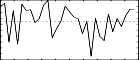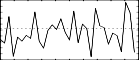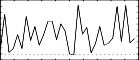## GEN21

GEN21 — Generates tables of different random distributions.

## Description

This generates tables of different random distributions. (See also betarand, bexprnd, cauchy, exprand, gauss, linrand, pcauchy, poisson, trirand, unirand, and weibull)

## Syntax

`f # time size 21 type level [arg1  [arg2]]`

## Initialization

time and size are the usual GEN function arguments. level defines the amplitude. Note that GEN21 is not self-normalizing as are most other GEN functions. type defines the distribution to be used as follow:

• 1 = Uniform (positive numbers only)

• 2 = Linear (positive numbers only)

• 3 = Triangular (positive and negative numbers)

• 4 = Exponential (positive numbers only)

• 5 = Biexponential (positive and negative numbers)

• 6 = Gaussian (positive and negative numbers)

• 7 = Cauchy (positive and negative numbers)

• 8 = Positive Cauchy (positive numbers only)

• 9 = Beta (positive numbers only)

• 10 = Weibull (positive numbers only)

• 11 = Poisson (positive numbers only)

Of all these cases only 9 (Beta) and 10 (Weibull) need extra arguments. Beta needs two arguments and Weibull one.

If type = 6, the random numbers in the ftable follow a normal distribution centered around 0.0 (mu = 0.0) with a variance (sigma) of level / 3.83. Thus more than 99.99% of the random values generated are in the range -level to +level. The default value for level is 1 (sigma = 0.261). If a mean value different of 0.0 is desired, this mean value has to be added to the generated numbers.

## Examples

```f1 0 1024 21 1       ; Uniform (white noise)
f1 0 1024 21 6       ; Gaussian (mu=0.0, sigma=1/3.83=0.261)
f1 0 1024 21 6 5.745 ; Gaussian (mu=0.0, sigma=5.745/3.83=1.5)
f1 0 1024 21 9 1 1 2 ; Beta (note that level precedes arguments)
f1 0 1024 21 10 1 2  ; Weibull```

All of the above additions were designed by the author between May and December 1994, under the supervision of Dr. Richard Boulanger.

Here is a complete example of the GEN21 routine. It uses the file gen21.csd.

Example 1287. Example of the GEN21 routine.

See the sections Real-time Audio and Command Line Flags for more information on using command line flags.

```<CsoundSynthesizer>
<CsOptions>
; Select audio/midi flags here according to platform
-odac  ;;;realtime audio out
; For Non-realtime ouput leave only the line below:
; -o gen21.wav -W ;;; for file output any platform
</CsOptions>
<CsInstruments>

sr = 44100
ksmps = 32
nchnls = 2
0dbfs  = 1

instr 1

ifn    = p4
isize  = ftlen(ifn)
prints "TABLE NUMBER: %d\n", ifn
prints "Index\tValue\n"

iindex = 0				;start loop
begin_loop:
ivalue tab_i iindex, ifn
prints "%d:\t%f\n", iindex, ivalue
iindex = iindex + 1
if (iindex < isize) igoto begin_loop

;produce sound - and repeat it 10 times so you can hear the patterns:
aphase phasor 10/10			;play all 32 values 10x over 10 seconds
aphase = aphase*isize			;step through table
afrq   table aphase, p4			;read table number
asig   poscil .5, (afrq*500)+1000,10	;scale values of table 500 times, add 1000 Hz
outs asig , asig			;so we can distinguish the different tables
endin

</CsInstruments>
<CsScore>
f1 0 32 21 1		;Uniform (white noise)
f2 0 32 21 6		;Gaussian (mu=0.0, sigma=1/3.83=0.261)
f3 0 32 21 6 5.745	;Gaussian (mu=0.0, sigma=5.745/3.83=1.5)
f4 0 32 21 9 1 1 2	;Beta (note that level precedes arguments)
f5 0 32 21 10 1 2	;Weibull
f10 0 8192 10 1		;Sine wave

i 1  0 10 1
i 1 11 10 2
i 1 22 10 3
i 1 33 10 4
i 1 44 10 5
e
</CsScore>
</CsoundSynthesizer>
```

These are the diagrams of the waveforms of the GEN21 routines, as used in the example:f 1 0 32 21 1 - positive numbers onlyf 2 0 32 21 6f 3 0 32 21 6 5.745f 4 0 32 21 9 1 1 2 - positive numbers onlyf 5 0 32 21 10 1 2 - positive numbers only

## Credits

 Author: Paris Smaragdis MIT, Cambridge 1995

 Author: John ffitch University of Bath/Codemist Ltd. Bath, UK

Precisions about mu and sigma added by François Pinot after a discussion with Joachim Heintz on the Csound List, December 2010.

New in Csound version 3.2